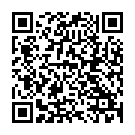## Add and Subtract on a Number Line

### Related Worksheets

Add and subtract near multiples on a number line. Develop mental methods of addition and subtraction by adding and subtracting a multiple of 10 or 100, and then adjusting. For example add 29 by adding 30 and then subtracting 1.